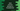# Java Program to convert decimal to Hexadecimal## Java program to convert a decimal number to Hexadecimal :

In this tutorial, I will show you how to convert a decimal number to Hexadecimal in Java using two different methods.

To convert a number from decimal to Hexadecimal :

1. Get the number from the user using Scanner
2. Pass it to a different method to find out the hexadecimal
3. First get the reminder dividing the number by 16
4. If it is less than 10, add it to a result string. The result string should be empty at first. We will keep updating the result each time.
5. If the remainder is greater than or equal to 10, add to the result string_ ‘A’,‘B’,‘C’,‘D’,‘E’ or ‘F’ for 10,11,12,13,14 and 15_ respectively.
6. Now, change the number to_ number/16_
7. Iterate it till the number is greater than 0

### Example Program :

``````import java.util.HashMap;
import java.util.Scanner;
import java.util.StringJoiner;

public class Test {

static char hexaChar[] = {'F','E','D','C','B','A'};

static String findHexa(int no){
String result =""; //variable to store final result
int reminder ; //variable to store reminder

while(no>0){
reminder = no % 16;

if(reminder > 9){
result = hexaChar[15 - reminder] + result;
}else{
result = reminder+result;
}
no = no/16;
}
return result;
}
public static void main(String[] args) {
Scanner scanner = new Scanner(System.in);

System.out.println("Enter a number ");
int num = scanner.nextInt();
System.out.println("Hexa "+findHexa(num));
}
}``````

### Output :

``````Enter a number
1234
Hexa 4D2

Enter a number
12365
Hexa 304D``````

We can also use public static String toHexString(int i) method of _Integer _class to convert a number to Hexadecimal directly in Java. Program :

``````import java.util.Scanner;

public class Test {
public static void main(String[] args) {
Scanner scanner = new Scanner(System.in);

System.out.println("Enter a number ");
int num = scanner.nextInt();
System.out.println("Hexa "+Integer.toHexString(num));
}
}``````

The output is same as the first example.